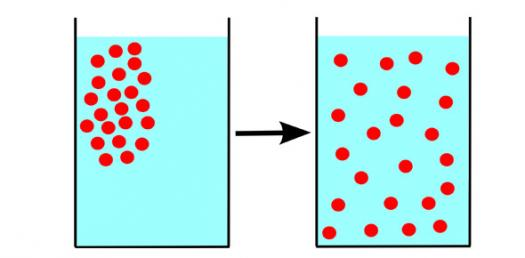# Lab 1 Diffusion And Osmosis Pre-lab Quiz

10 Questions | Total Attempts: 2335SettingsLab 1 diffusion and osmosis pre-lab quiz for coach wimpey and coach johnson.

• 1.
What is the purpose of IKI in the lab?
• A.

To indicate the presence of glucose

• B.

To react with the dialysis tubing

• C.

To indicate the presence of starch

• D.

To color the solution red

• 2.
In this lab the dialysis tubing represents
• A.

Mitochondria

• B.

Plasma Membrane

• C.

Chloroplast

• D.

Nucleus

• 3.
Which of the following is true of sucrose?
• A.

It is non-polar

• B.

It is table sugar

• C.

It is the same as starch

• D.

It will not dissolve in water

• 4.
Which molecule is larger, glucose or IKI?
• A.

IKI

• B.

Glucose

• C.

Can't tell

• 5.
A 0.2 M solution of sucrose will have
• A.

.2 molecules of sucrose per milliliter of water

• B.

.2 moles of sucrose per milliliter of water

• C.

.2 moles of sucrose per L of water

• D.

.2 moles of sucrose per L of solution

• 6.
Which solution list below is most hypertonic relative to distilled water?
• A.

0.0 M sucrose

• B.

0.2 M sucrose

• C.

0.4 M sucrose

• D.

0.6 M sucrose

• 7.
How is the presence of glucose detected?
• A.

Glucose test strip

• B.

IKI

• C.

Change in mass

• D.

All of the above

• 8.
In the lab how can you tell whether the dialysis bags gained or lost water?
• A.

By the change in color

• B.

By the change in volume

• C.

By the change in mass

• D.

You cannot tell

• 9.
Osmosis is
• A.

The diffusion of sucrose

• B.

The diffusion of IKI

• C.

The diffusion of glucose

• D.

The diffusion of water

• 10.
How does water move?
• A.

Always from higher potential to lower potential

• B.

Always from lower potential to higher potential

• C.

Movement varies depending on mass

• D.

Cannot tell until you do the lab

Related TopicsBack to top